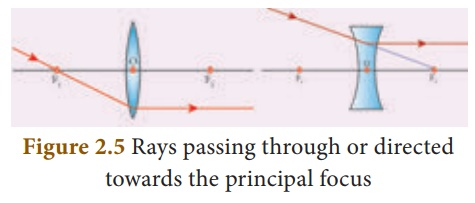Home | | Science 10th Std | Images Formed Due to Refraction Through a Convex and Concave Lens

# Images Formed Due to Refraction Through a Convex and Concave Lens

When an object is placed in front of a lens, the light rays from the object fall on the lens. The position, size and nature of the image formed can be understood only if we know certain basic rules.

IMAGES FORMED DUE TO REFRACTION THROUGH A CONVEX AND CONCAVE LENS

When an object is placed in front of a lens, the light rays from the object fall on the lens. The position, size and nature of the image formed can be understood only if we know certain basic rules.

Rule- 1: When a ray of light strikes the convex or concave lens obliquely at its optical centre, it continues to follow its path without any deviation (Figure 2.3).Rule-2: When rays parallel to the principal axis strikes a convex or concave lens, the refracted rays are converged to (convex lens) or appear to diverge from (concave lens) the principal focus (Figure 2.4).Rule-3: When a ray passing through (convex lens) or directed towards (concave lens) the principal focus strikes a convex or concave lens, the refracted ray will be parallel to the principal axis (Figure 2.5).Study Material, Lecturing Notes, Assignment, Reference, Wiki description explanation, brief detail
10th Science : Chapter 2 : Optics : Images Formed Due to Refraction Through a Convex and Concave Lens |